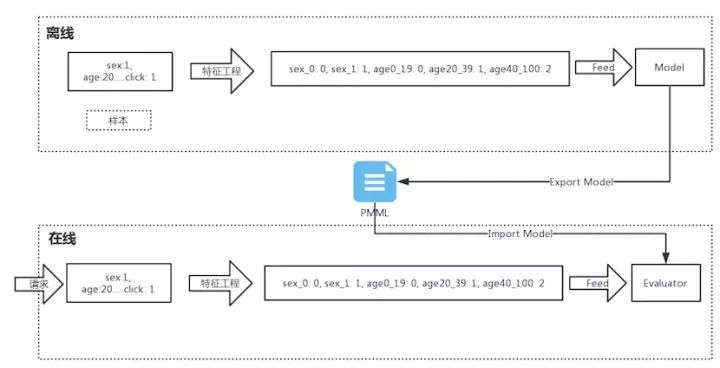>科技>正文

# 如何使用 Java 调取 Python、R 的训练模型？PMML

PMML 是 Predictive Model Markup Language 的缩写，翻译为中文就是“预测模型标记语言”。它是一种基于XML的标准语言，用于表达数据挖掘模型，可以用来在不同的应用程序中交换模型。

PMML 能做什么• 整个流程分为两部分：离线和在线。
• 离线部分流程是将样本进行特征工程，然后进行训练、生成模型。一般离线部分常用 Python 中的 sklearn、R 或者 Spark ML 来训练模型。
• 在线部分是根据请求得到样本数据，对这些数据采用与离线特征工程一样的方式来处理，然后使用模型进行评估。一般在线部分常用 Java、C++ 来开发。
• 离线部分与在线部分是通过 PMML 连接的，也就是说离线训练好了模型之后，将模型导出为 PMML 文件，在线部分加载该 PMML 文件生成对应的评估模型。

pip install --user git+https://github.com/jpmml/sklearn2pmml.git

fromsklearn.ensemble importRandomForestClassifier

fromsklearn2pmml importPMMLPipeline, sklearn2pmml

# 创建带有特征名称的 DataFrame

iris_df = pd.DataFrame(iris.data, columns=iris.feature_names)

# 创建模型管道

iris_pipeline = PMMLPipeline([

( "classifier", RandomForestClassifier())

])

# 训练模型

iris_pipeline.fit(iris_df, iris.target)

# 导出模型到 RandomForestClassifier_Iris.pmml 文件

sklearn2pmml(iris_pipeline, "RandomForestClassifier_Iris.pmml")

<dependency>

<groupId>org.jpmml </groupId>

<artifactId>pmml-evaluator </artifactId>

<version>1.4.1 </version>

</dependency>

<dependency>

<groupId>org.jpmml </groupId>

<artifactId>pmml-evaluator-extension </artifactId>

<version>1.4.1 </version>

</dependency>

importorg.dmg.pmml.FieldName;

importorg.dmg.pmml.PMML;

importorg.jpmml.evaluator.*;

importorg.jpmml.model.PMMLUtil;

importorg.xml.sax.SAXException;

importjavax.xml.bind.JAXBException;

importjava.io.FileInputStream;

importjava.io.FileNotFoundException;

importjava.io.IOException;

importjava.io.InputStream;

importjava.util.ArrayList;

importjava.util.HashMap;

importjava.util.List;

importjava.util.Map;

publicclassClassificationModel{

privateEvaluator modelEvaluator;

/**

* 通过传入 PMML 文件路径来生成机器学习模型

*

* @parampmmlFileName pmml 文件路径

*/

publicClassificationModel(String pmmlFileName){

PMML pmml = null;

try{

if(pmmlFileName != null) {

InputStream is = newFileInputStream(pmmlFileName);

pmml = PMMLUtil.unmarshal(is);

try{

is.close();

} catch(IOException e) {

System.out.println( "InputStream close error!");

}

ModelEvaluatorFactory modelEvaluatorFactory = ModelEvaluatorFactory.newInstance();

this.modelEvaluator = (Evaluator) modelEvaluatorFactory.newModelEvaluator(pmml);

modelEvaluator.verify();

System.out.println( "加载模型成功！");

}

} catch(SAXException e) {

e.printStackTrace();

} catch(JAXBException e) {

e.printStackTrace();

} catch(FileNotFoundException e) {

e.printStackTrace();

}

}

// 获取模型需要的特征名称

publicList<String> getFeatureNames(){

List<String> featureNames = newArrayList<String>();

List<InputField> inputFields = modelEvaluator.getInputFields();

for(InputField inputField : inputFields) {

}

returnfeatureNames;

}

// 获取目标字段名称

publicString getTargetName(){

returnmodelEvaluator.getTargetFields().get( 0).getName().toString();

}

// 使用模型生成概率分布

privateProbabilityDistribution getProbabilityDistribution(Map<FieldName, ?> arguments){

Map<FieldName, ?> evaluateResult = modelEvaluator.evaluate(arguments);

FieldName fieldName = newFieldName(getTargetName());

return(ProbabilityDistribution) evaluateResult.get(fieldName);

}

// 预测不同分类的概率

publicValueMap<String, Number> predictProba(Map<FieldName, Number> arguments){

ProbabilityDistribution probabilityDistribution = getProbabilityDistribution(arguments);

returnprobabilityDistribution.getValues();

}

// 预测结果分类

publicObject predict(Map<FieldName, ?> arguments){

ProbabilityDistribution probabilityDistribution = getProbabilityDistribution(arguments);

returnprobabilityDistribution.getPrediction();

}

publicstaticvoidmain(String[] args){

ClassificationModel clf = newClassificationModel( "RandomForestClassifier_Iris.pmml");

List<String> featureNames = clf.getFeatureNames();

System.out.println( "feature: "+ featureNames);

// 构建待预测数据

Map<FieldName, Number> waitPreSample = newHashMap<>();

waitPreSample.put( newFieldName( "sepal length (cm)"), 10);

waitPreSample.put( newFieldName( "sepal width (cm)"), 1);

waitPreSample.put( newFieldName( "petal length (cm)"), 3);

waitPreSample.put( newFieldName( "petal width (cm)"), 2);

System.out.println( "waitPreSample predict result: "+ clf.predict(waitPreSample).toString());

System.out.println( "waitPreSample predictProba result: "+ clf.predictProba(waitPreSample).toString());

}

}

feature: [sepal length (cm), petal width (cm), sepal width (cm), petal length (cm)]

waitPreSample predict result: 1

waitPreSample predictProba result: {0=0.0, 1=0.5, 2=0.5}